Jiang, D., Zhang, Y., and Chen, J. (2023). “Non-destructive testing of mechanical properties of solid wood panel based on partial least squares structural equation modeling transfer method,” BioResources 18(2), 3620-3641.

#### Abstract

Calibration transfer between near infrared (NIR) spectrometers is a subtle issue in the chemometrics and process industry. Similar instruments may generate strongly different spectral responses, and regression models developed on a first NIR system can rarely be used with spectra collected by a second apparatus. In this work, two novel methods based on Structural Equation Modeling (SEM), called Enhanced Feature Extraction Approaches for factor analysis (EFEA-FA) and Enhanced Feature Extraction Approaches for spectral space transformation (EFEA-SST), were proposed to perform calibration transfer between NIR spectrometers. They were applied to a NIR nondestructive testing model for solid wood panels mechanical properties. Four different standardization algorithms were evaluated for transferring solid wood panels quality databases between a portable NIRS (InGaAs)-array spectrometer (NIRquest512) and a HSI Camera (SPECIM FX17). The results showed that EFEA-SST yielded the best model evaluation metrics (R2 and Root Mean Square Error of Prediction (RMSEP)) values for tensile strength (RMSEP=11.309, R2=0.865) parameters, while EFEA-FA gave the best fit for flexural strength (RMSEP=10.653, R2=0. 912). These results suggest the potential of two novel quality parameters prediction methods based on spectral databases transferred between diverse NIRS spectrometers.

Non-Destructive Testing of Mechanical Properties of Solid Wood Panel Based on Partial Least Squares Structural Equation Modeling Transfer Method

Dapeng Jiang,a Yizhuo Zhang,a,* and Chen Jinhao b

Calibration transfer between near infrared (NIR) spectrometers is a subtle issue in the chemometrics and process industry. Similar instruments may generate strongly different spectral responses, and regression models developed on a first NIR system can rarely be used with spectra collected by a second apparatus. In this work, two novel methods based on Structural Equation Modeling (SEM), called Enhanced Feature Extraction Approaches for factor analysis (EFEA-FA) and Enhanced Feature Extraction Approaches for spectral space transformation (EFEA-SST), were proposed to perform calibration transfer between NIR spectrometers. They were applied to a NIR nondestructive testing model for solid wood panels mechanical properties. Four different standardization algorithms were evaluated for transferring solid wood panels quality databases between a portable NIRS (InGaAs)-array spectrometer (NIRquest512) and a HSI Camera (SPECIM FX17). The results showed that EFEA-SST yielded the best model evaluation metrics (R2 and Root Mean Square Error of Prediction (RMSEP)) values for tensile strength (RMSEP=11.309, R2=0.865) parameters, while EFEA-FA gave the best fit for flexural strength (RMSEP=10.653, R2=0. 912). These results suggest the potential of two novel quality parameters prediction methods based on spectral databases transferred between diverse NIRS spectrometers.

DOI: 10.15376/biores.18.2.3620-3641

Keywords: Solid wood panel; Calibration transfer; Near infrared; Hyperspectral; Mechanical property

Contact information: a: College of Computer Science and Artificial Intelligence, Changzhou University, 1 gehu middle Rd., Changzhou, 213164, China; b: College of Mechanical and Electrical Engineering, Northeast Forestry University, 26 Hexing Rd., Harbin, 150040, China;

* Corresponding author: nefuzyz@163.com

INTRODUCTION

The forest products industry utilizes near infrared spectroscopy (NIRS) for quantitative examination of solid wood panels, such as tensile strength and flexural strength. NIRS offers just the mean spectrum of a sample (Tuncer 2022), regardless of the scanned area of the sample. As a result of averaging the gathered spectra to produce a single spectrum, information regarding the spatial distribution of constituents inside the sample is lost. The development of NIR hyperspectral imaging (HSI), combines NIR spectroscopy with digital imaging (Lima et al. 2022; Yakubu et al. 2022). For each pixel in the imaging plane, hyperspectral pictures can be collected spanning the whole visible and NIR wavelength range of a material (Vidal and Pasquini 2021). Consequently, using this stack of wavelength images or spectral cube, the average intensity and local changes of the intensity pixels at each spectral image may be analyzed and used for pattern identification. However, expensive and specialized hardware is required to capture hyperspectral images (Nakawajana et al. 2021; Tunny et al. 2022). In general, hyperspectrometers with somewhat higher resolution cost over a million dollars. To solve this problem, calibration transfer technology strikes a balance between price and resolution, and near-infrared spectrum transfer technology was used to transfer the calibration model from the high-precision near-infrared spectrometer equipment platform in the laboratory to the low-precision hyperspectral equipment (Wang et al. 2023). A high-precision detection model derived from a laboratory NIR spectrometer was transferred to an industrial-grade online HSI spectrometer to improve the model’s accuracy and reduce the overhead of the industrial pipeline.

Several standardisation approaches address this crucial issue and permit the transfer of calibration models (X. Li et al. 2021). There are methods for resolving transfer problems that do not require standardization (multiplicative scatter correction (MSC), orthogonal signal correction (OSC), etc.) (Shan et al. 2020). However, when the issue is not caused by spectral intensity variations but rather by wavelength shifts, various standardization approaches might be utilized. According to Qiao et al. (2021), a calibration transfer can be performed in a few ways: a priori correction involves correcting the spectra prior to applying the existing calibration model; model correction involves adapting the calibration model; and a posteriori correction involves correcting the predictions of the existing calibration model. Some modern transfer techniques are based on factor analysis, which separates spectral information related elements from noise to enhance transfer outcomes.

In the framework of transfer between instruments, a priori correction and model correction are based on multivariate spectrum correction. In the first mode, secondary spectra are matched to primary spectra and entered the existing model. In the second mode, the spectra of the primary spectra database are adjusted to match those of the secondary database, and the model is recalibrated. Spectra multivariate correction may use a large number of techniques, such as direct standardisation (DS) (Tian et al. 2022), or piecewise direct standardisation (PDS) (Sun et al. 2021; Chen et al. 2022). In a posteriori correction, existing primary spectra are applied to secondary spectra whose responses are known. After calibrating a model of the prediction error, its inverse is used to make future predictions. Typically, a simple univariate technique, such as bias/slope correction (BSC) of the projected values (Salguero-Chaparro et al. 2013), is used to implement this model.

Factor analysis (FA) is a highly effective method for establishing relationships between two sets of measurements (spectrum of two instruments). Transfer methods based on factor analysis, such as the Spectral Space Transformation algorithm (SST) (L. Li et al. 2022; Du et al. 2011), the alternating trilinear decomposition (ATLD) algorithm (Yap et al. 2022), the Principal Component Analysis (PCA) algorithm (Rehman et al. 2022), and the Canonical Correlation Analysis (CCA) algorithm (Fan et al. 2008; Zheng et al. 2014), have been widely applied and are frequently compared to traditional methods. FA, in contrast to PCR or PLS (Mendoza et al. 2018), utilizes correlation rather than covariance. A substantial covariance between two instruments NIR spectrum is not always indicative of a significant connection. A pair of those spectrum may have perfect correlation but low covariance. In such instances, correlation (FA) should be utilized rather than covariance (PCR or PLS).

PCA algorithm, CCA algorithm, and ATLD algorithm are statistically classified as exploratory factor analysis (EFA) approaches. In addition, the succession of peaks and troughs that show in an NIR spectrum represent the sample’s molecular vibrations. The relationship between molecular overtones and combined bands in the NIR spectrum and the stretching vibrations of hydrocarbon bonds in solid wood panels is linear. By comparing the NIR spectra of wood and pure chemical components such as lignin, cellulose, extractives, and xylan, researchers estimate the contribution of the chemical components to the mechanical performance of wood, in accordance with the premise of Confirmatory Factor Analysis (CFA) (Dash and Paul 2021). CFA assesses a priori hypotheses derived from earlier research on the link between the mechanical characteristics of solid wood panels and conducts validated testing on proposed models.

Lignin content and the lignin and extractives are strongly correlated with the absorbances near 1668 nm and 1684 nm (Horvath et al. 2011). The microfibril angle of wood samples were correlated with the absorbances near 1,150 nm. The absorbance peaks between 1075 and 1250 nm had been identified to be related to the lignin content (Watanabe et al. 2012). The band at 1143 nm belonged to aromatic groups, and the absorbances near 1130 nm were closely related to the lignin content after 2-d preprocess. Fujimoto et al. (2012) believed that the absorbances near 1428.5 nm were highly correlated with cellulose, the bands at 1366 nm, 1400 nm , and 1428 nm were associated with the cellulose and the moisture content, the band at 1396.6 nm was highly correlated with freewater, and the band at 1366.1 nm was highly correlated with freewater. The bands are highly correlated with bound water. Therefore, it is feasible to hypothesize, in advance, the number of factors affected the mechanical properties of wood, whether these factors are correlated, and which band in NIR load onto and reflect which factors, according to the above literature.

CFA is utilized by Partial Least Squares Structural Equation Modeling (PLS-SEM) (Sarstedt et al. 2022; Smith et al. 2022) to evaluate the measurement model. It differs from EFA in that it evaluates using empirical data with existing factor specification. For CFA, model fit is examined to validate the measurement. After the model is fitted, the route models between the latent variables are evaluated (Jonckere and Rosseel 2022). The PLS-SEM approach is intriguing because it allows them to estimate complicated models with numerous constructs, indicator variables, and structural routes without putting distributional assumptions on the data (Hair et al. 2020). PLS-SEM is a causal-predictive approach to SEM that stresses prediction in estimating statistical models, the structures of which are aimed to give causal explanations (Hult et al. 2021). Thus, the method avoids the seeming conflict between explanation and prediction, which is normally stressed in academic research. In addition, user-friendly software packages are available, such as PLS-Graph (Tenenhaus and Hanafi 2010) and SmartPLS (Sarstedt and Cheah 2019), that need minimum technical knowledge of the method.

In addition to introducing the CFA theory into the NIR transfer model, the purpose of this study is to present a unified calibration transfer framework based on the PLS-SEM model. Based on this framework, two existing algorithms, CCA and SST, were unified into a new process, named EFEA-FA and EFEA-SST. This work introduces Structural Equation Modeling in statistics and improves model transfer methods from a novel perspective. The band is selected according to the fundamentals of NIR spectroscopy. Using EFEA-FA and EFEA-SST for the prediction of the Solid Wood Panels mechanical character could reach great performance over both source and target domain. This work determined the number of factors and the relationship between factors and spectral bands. These components were chosen in accordance with knowledge from previous research as well as the PLS-SEM model’s unified structure. The goal was to improve the interpretability of the transfer model.

EXPERIMENTAL

Materials

Logs went through the initial wood working process, for instance peeling or debarking. Next, wood was cut and processed into solid wood panels. A set of 90 Sheets of solid wood panels samples were processed. In the lab, the solid wood boards were kept at 5 °C and 90% relative humidity. Before spectroscopic measurements, the samples were equilibrated to room temperature (25 °C).

Spectra were collected from samples in reflectance mode (log 1/R) using two NIRS-instruments, and 2 batches of NIR samples were established: (1) portable NIRS (InGaAs)-array spectrometer (NIRquest512, Orlando, FL, USA) and (2) HSI Camera (SPECIM FX17, Oulu, Finland). The Smart PLS (3.2.8) statistical tool was used to examine the data through partial least square equation modeling (PLS-SEM), and MATLAB, a proprietary multi-paradigm programming language and numeric computing environment developed by MathWorks, was used to propose a novel model to achieve calibration transfer.

Figure 1 illustrates the HSI camera. A line-scan mode SPECIM FX17 provided with a transport module was used to measure all spectra in the scanning range of 900 to 1700 nm, at 8 nm intervals. A rectangular natural product cell with a window surface of 94.9 cm2 was used. Each spectrum was the average of 32 scans. Spectra were captured using the CameraLink and GigE Vision Camera Interface in conjunction with the LUMO Software Development Kit (LUMO Development Kit, Oulu, Finland).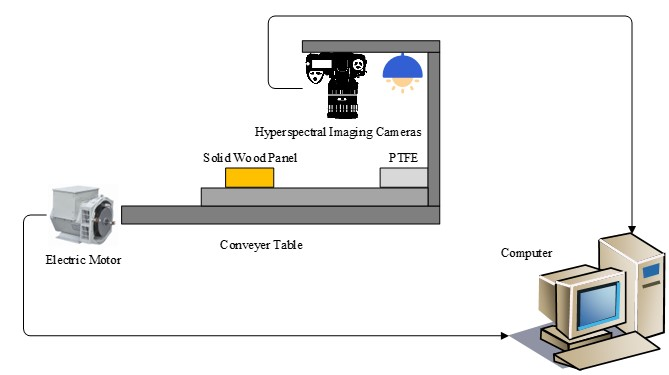Fig. 1. SPECIM FX17 Camera

Figure 2 shows the NIRS Spectrometer. The second instrument was a NIRQuest512 spectrometer based on an array detector. The spectrometer with an InGaAs array work in the range from 900 to 1700 nm, with a spectral wavelength interval of 3.1 nm. The distance from the sample surface to the sensing head was approximately 13 mm. With an integration time of 5 s, 10 scans were averaged for each measurement. A total of 30 spectra of each sample were acquired, and the mean spectrum was used for data processing. All spectra were recorded using SpectraSuite 2.0 (Quadrangle Blvd, Orlando, FL, USA).

This study focused on the restoration and maintenance of ancient wooden structures, specifically the ancient bucket arch structures found in China. In analyzing these structures, it is equally important to consider both their ultimate strength index and their stiffness index. The study focused on the wood strength both under flexural and tension stresses. The mechanical testing material used in this study was birch wood grown in Northeast China, which was collected from the Chonghe Forest Farm of the Forestry Bureau of Wuchang City, Heilongjiang Province. The forest farm is characterized by continuous mountains, criss-crossing terrain, high elevation in the east and low elevation in the west, a dense river network, and abundant water sources. The geographical location and environmental conditions are detailed in Table 1.

Table 1. Climatic Conditions of Log Collection Areas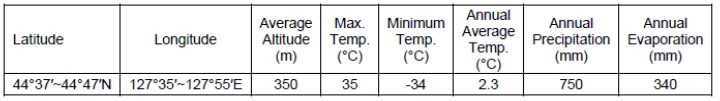Within the birch forest, three groups of sample trees were selected based on their elevation, with each group containing four trees for a total of 12 sample trees. The trees were 20 years old, with a height of 12 to 14 m and a diameter at breast height of 16 to 17 cm. After marking the growth direction of each tree, the sample logs were felled, and the logs were cut at chest height (about 1.3 m above the ground). To highlight the differences in mechanical properties of the test materials, interval sampling was adopted during cutting to account for the distribution characteristics of mechanical properties in the vertical direction. Specifically, wood sections with a length of 1 m were cut for processing the bending test materials, while wood sections with a length of 2 m were cut for processing the tensile test materials, as shown in Fig. 2.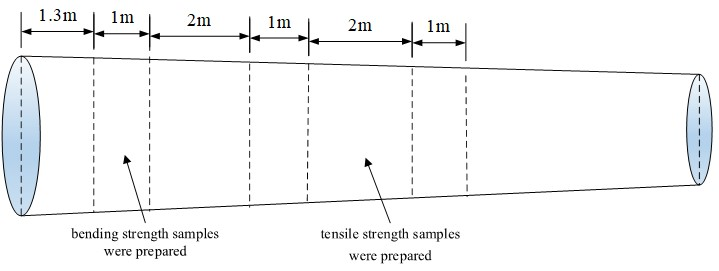Fig. 2. Schematic diagram of wood cutting process

After air-drying, the wood section was sawn, excluding the pithwood and without distinguishing between the heartwood and sapwood. First, rough strips for bending resistance were made, and then bending strength values of samples of 300 mm × 20 mm × 20 mm were prepared in accordance with the Chinese National Standard (GB/T 1928-2009). Out of the total samples, 90 flawless samples were selected. These were numbered from 1 to 90, and placed in a drying oven to reduce moisture content to 12%. To prevent moisture from affecting the samples, each one was placed in an airtight plastic bag. Reference values for flexural strength and tensile strength in solid wood panels samples were determined using official analysis methods. On solid wood panel samples, flexural strength was determined using the method of testing in bending strength of wood procedure (GB 1936.1-2009), which was proposed by the Standardization Administration of China (SAC, 2003). The tensile strength parallel to grain of wood (GB/T 1938.1-2009) was used to determine tensile strength.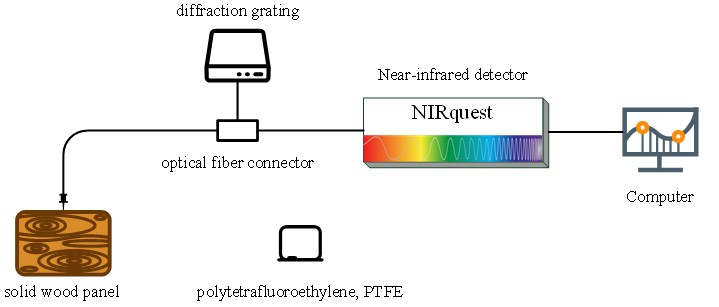Fig. 3. NIR Quest 512 Spectrometer

Source instrument and target instrument matrices are used to denote the primary and secondary spectra. After preprocessing spectral data, 90 samples were obtained from each instrument. The source domain dataset Xsource (90×512), target domain dataset Xtarget (90×224), and Y (90×2), were divided into three groups: transfer (Xt, Yt), calibration (Xc, Yc) and prediction (Xp, Yp), in the following proportions: 60% (54 samples), 10% (9 samples) and 30% (27 samples), respectively. The transfer set, calibration set, and prediction set of source spectra are denoted by Xst, Xsc, and Xsp, while the analogous sets of target spectra are denoted by Xtt, Xtc, and Xtp. The parameter Xtnew represents the spectra corrected from Xpt by calibration transfer, whereas ys and yt represent the sample concentrations that correspond to the source and target spectra, respectively. In this article, the values of ys and yt are the same.

Calibration Transfer Based on CCA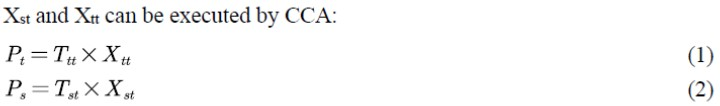where Tst and Ttt stand for the canonical weights, while Ps and Pt indicate the corresponding canonical scores of Xst and Xtt, respectively. The transfer matrix T was computed as follows,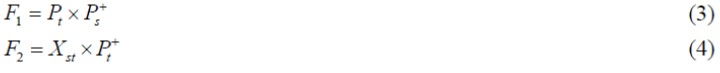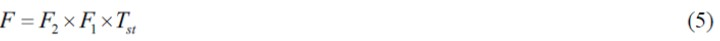where superscript “+” represents the pseudo-inverse, and both F1 and F2 are interim matrices for calculating F. Next, the Xtnew corrected from Xsp can be obtained by right multiplying F.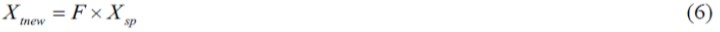Finally, substituting Bnew into the calibration model of Ac can yield the predicted values directly.

EFEA-FA Modeling

Generalizing: The PLS-SEM modeling and relationship with the wood biological function

PLS-SEM is a statistical technique that bears some resemblance to principal components analysis; however, instead of finding hyper-planes of maximum variance between the response and independent variables, it includes a method for assessing measurement model quality known as CFA. Researchers have began referring to the measurement model assessment stage in PLS-SEM as CCA in recent years (Henseler et al. 2014; Schuberth et al. 2018). CCA is a methodical procedure for systematically validating measurement models in PLS-SEM.

When conducting CCA with formative composite measurement models, the formative composite measurement models are linear combinations of the construct’s indicators. The indicators are deemed causal and do not always co-vary, pointing from the measured variables to the composite concept. Therefore, the internal consistency principles underlying reflective measurement models cannot be applied to formative measurement models.

Due to its oriented fibers, wood has been viewed as an anisotropic material. The tensile strength of wood has a high correlation with the fiber angle, whereas the tensile strength of wood along the grain direction is more dependent on the fiber angle and the strength of bundles of molecular chains that combine in groups to form the cellulose fibres; the flexural strength of wood is largely a function of lignin. Lignin, a crucial structural component in the supporting tissues of most plants, is a reliable predictor of flexural strength and stiffness.

When the SEM is based on secondary data, a reflective or formative secondary measure of the same construct should be identified and used as a proxy variable for assessing the convergent validity of formative constructs. It is possible to identify acceptable endogenous reflectively assessed items for use as proxy variables in convergent validity testing by analyzing established scales from prior research. We chose three variables for this study: lignocellulose, moisture content, and lignin. This study selected as observation variables from the 900 nm to 1700 nm spectral bands 1668 nm to 1684 nm, 1075 nm to 1250 nm, 1070 nm to 1150 nm, 1366 nm, 1400 nm, and 1428 nm, based on previous research on the link between NIR bands and wood strength. Utilizing three variables as latent variable factors. The path dependence between latent variable factors (lignin, etc.) and observable variables (spectral bands) is established based on past research findings, and a PLS-SEM structural equation model is created.

EFEA-FA modeling steps

The EFEA-FA model is a new regression model designed by the PLS-SEM structural. As a statistical method that bears some resemblance to principal components analysis, rather than finding hyper-planes of maximum variance between the Mechanical properties of wood and the near infrared wavelength spectrum, PLS-SEM computes latent variables from linear combinations of sets of specific wavelengths that correspond to previous research results to represent the Concept of wood Biological Functions. Based on the PLS-SEM calculated factors or composites, we developed the NIR spectral factor analysis transfer model.

The measuring model and the structural model make up SEM. A measurement model measures latent variables or composite variables, but a structural model examines all conceivable dependencies using path analysis. This is how the measurement model is expressed: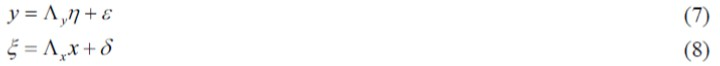where y is the dependent variable, for this study, y is the measured value of Mechanical properties for wood; Where x is the independent variable, for this study, x is the measured value of NIR for wood;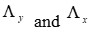are PLS-SEM loadings matrices;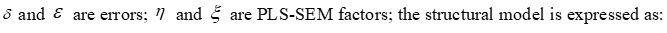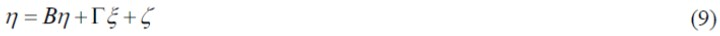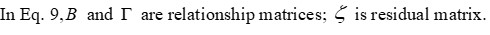According to approximate estimation and iterative optimization of EM method, the optimal solution of PLS-SEM relationship matrix and factors is obtained. The simplified flow chart of the model is as follows: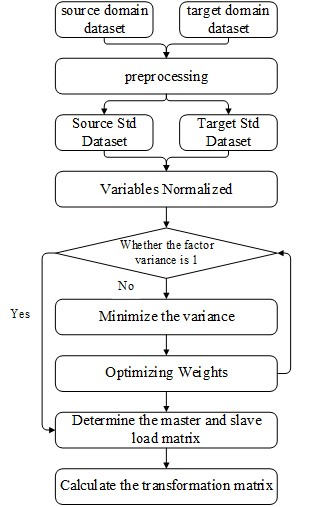Fig. 4. PLS-SEM Framework Flowchart

Step 1: Establishing the PLS-SEM path model of wood mechanical properties.

Step 2: Iterating PLS-SEM model to optimize the relationship matrix, latent variables and parameters, the algorithm including the apparent variables normalized, the loading matrix approximation estimation, the relationship matrix approximation estimation and weight estimation.

The apparent variables normalized

The NIR spectral band and the mechanical properties of solid wood panels are normalized: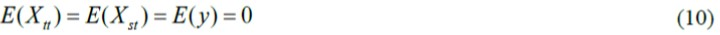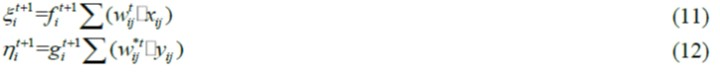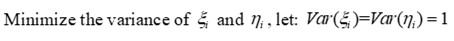The relationship matrix approximation estimation

Approximate Estimation of Relationship Matrix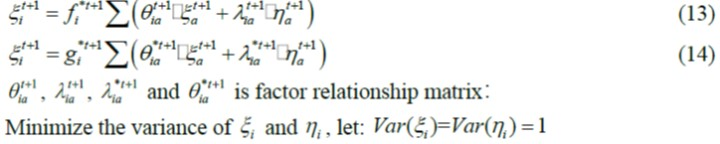Weight estimation

Iterative results of load matrix and score matrix for PLS-SEM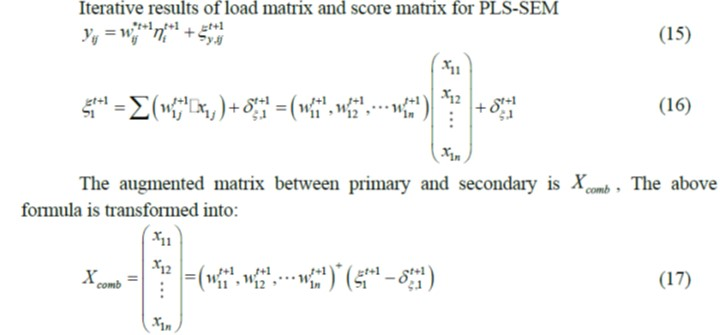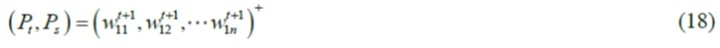The score matrix Ttt of the host spectrum and the score matrix Tst of the secondary spectrum are expressed as: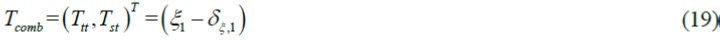Step 3: Calculate the conversion matrix T  between T<sub>st</sub> and T<sub>tt</sub> and transfer the spectral matrix Xsp from the machine to Xtnew. The formula is as follows: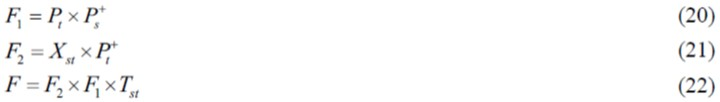Here, superscript “+” represents the pseudo-inverse, and both F1 and F2 are interim matrices for calculating F. Next, the Xtnew corrected from Xsp can be obtained by right multiplying F.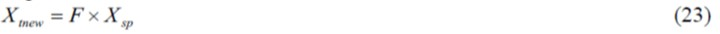Finally, substituting Xtnew into the calibration model can yield the predicted values directly.

In summary, the algorithm block diagram of EFEA-FA is shown in Fig. 5: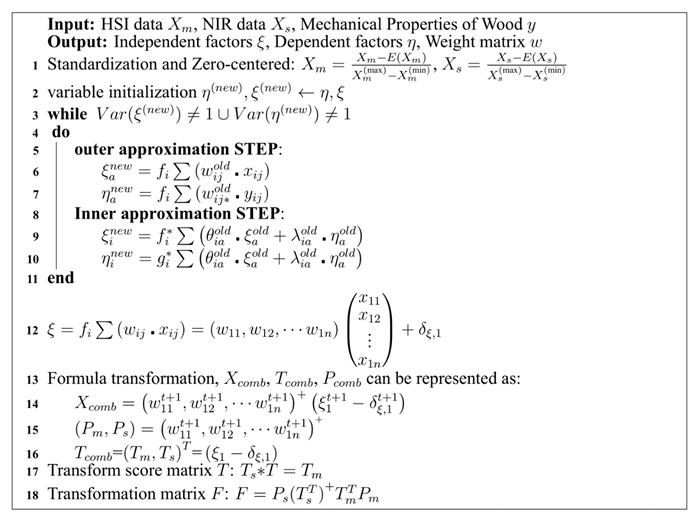Fig. 5. EFEA-FA Algorithm

Calibration Transfer Based on SST

Assume the rows of the spectral matrices Xtt and Xst are the corresponding spectra of the same subset of standardization samples measured on the primary and secondary instruments (or under the initial calibration and the modified test conditions), respectively. Let the singular value decomposition of Xcomb as follows: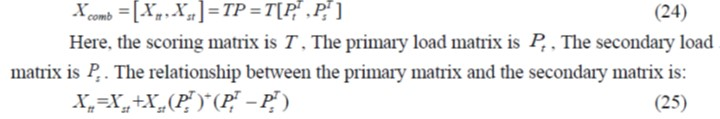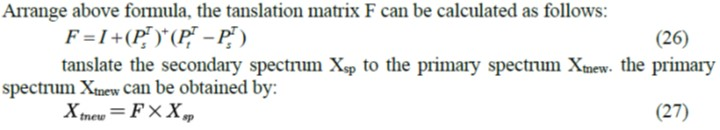EFEA-SST Modeling

The second model in our paper is EFEA-SST used for source domain data and target domain data respectively. As well as EFEA-FA, the EFEA-SST also implement calibration transfer based on the PLS-SEM framework. The specific algorithm flow is as follows:

Step 1: Establishing the PLS-SEM path model of wood mechanical properties.

Step 2: iterating PLS-SEM model to optimize the relationship matrix, latent variables and parameters, the algorithm including the apparent variables normalized, the loading matrix approximation estimation, the relationship matrix approximation estimation and weight estimation.

The apparent variables normalized

The NIR spectral band and the mechanical properties of solid wood panels are normalized: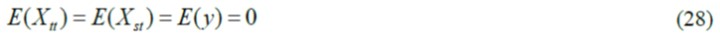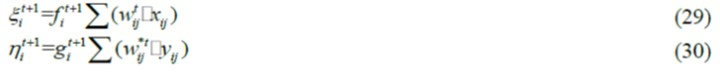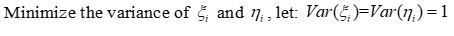The relationship matrix approximation estimation

Approximate Estimation of Relationship Matrix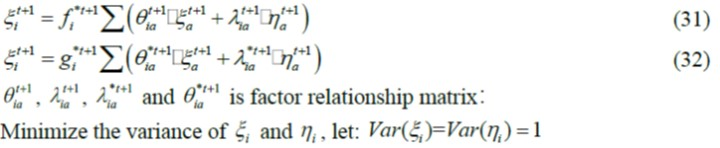Weight estimation

Iterative results of load matrix and score matrix for PLS-SEM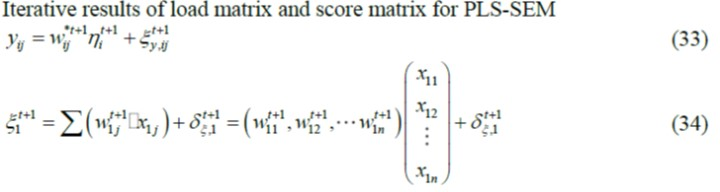The augmented matrix between primary and secondary is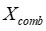, The above formula is transformed into: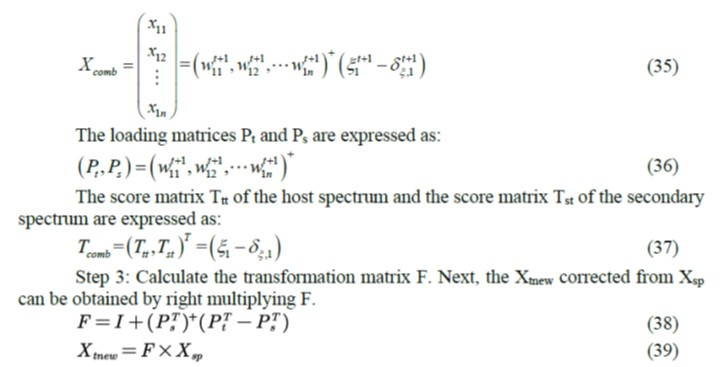In summary, the algorithm block diagram of EFEA-FA is shown in Fig. 6: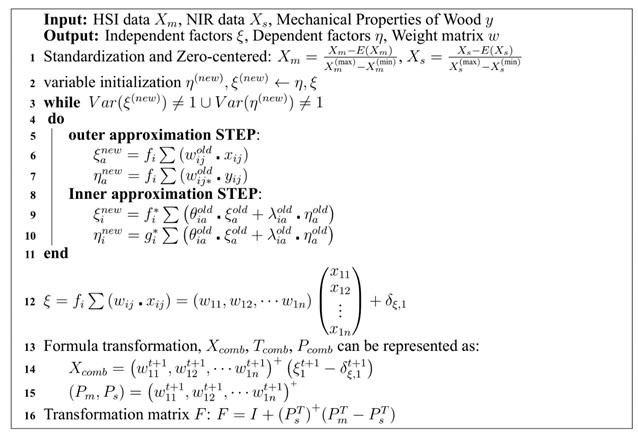Fig. 6. EFEA- SST Algorithm

RESULTS AND DISCUSSION

NIR Spectral Preprocessing Results

The raw spectra from the original database as measured by the NIRquest512 (Fig. 2) and the SPECIM FX17 (Fig. 1) spectrometers are depicted in Fig. 7 and Fig. 8, respectively. Some major bands were observed on both figures. It is important that the light scattering has a significant impact on the spectra collected by both instruments. In fact, a significant proportion of photons that are not caught by the sensors and are so assumed to be absorbed are multiplicative scattered. After the log transform, this is additive scattered.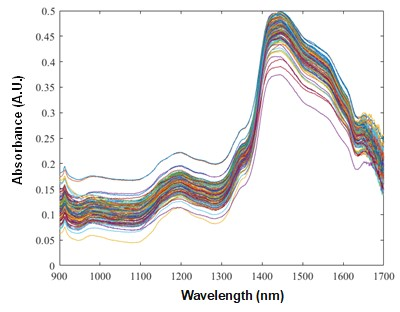Fig. 7. Unprocessed primary spectra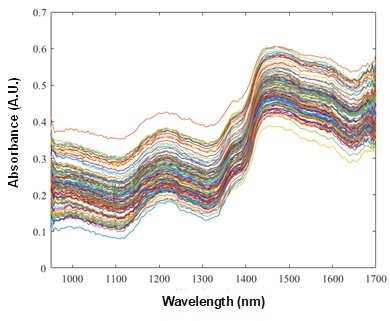Fig. 8. Unprocessed secondary spectra

In this experiment, we used two classical spectrum preprocessing methods, standard normal variate (SNV) and Savitzky Golay Filter (S-G), to identify the spectral preprocessing approach with the greatest generalization capability. For each spectrum preprocessing approach, we first applied the identical spectral preprocessing to the source domain and the target domain, then used the processed source domain to create the calibration transfer models and the processed source domain and target domain to evaluate the performance. As depicted in Figs. 9-12, the spectra of each dataset were drawn following SNV-SG spectral preprocessing. Compared to Figs. 7, 8, The spectral gaps between the datasets were substantially decreased, and the spectral signal was noticeably smoothed.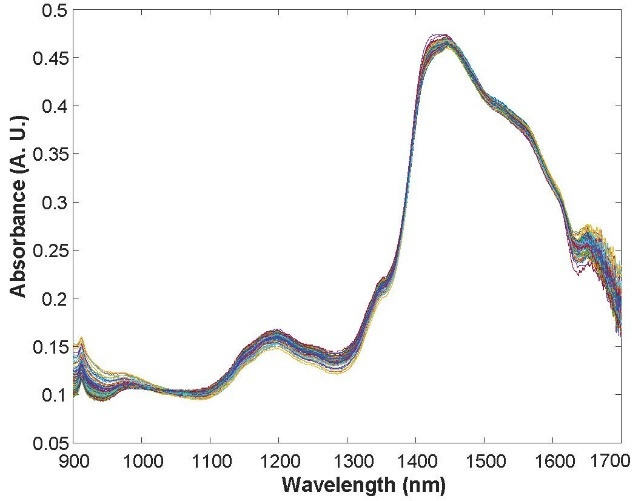Fig. 9. Primary spectra pre-processed using the SNV method